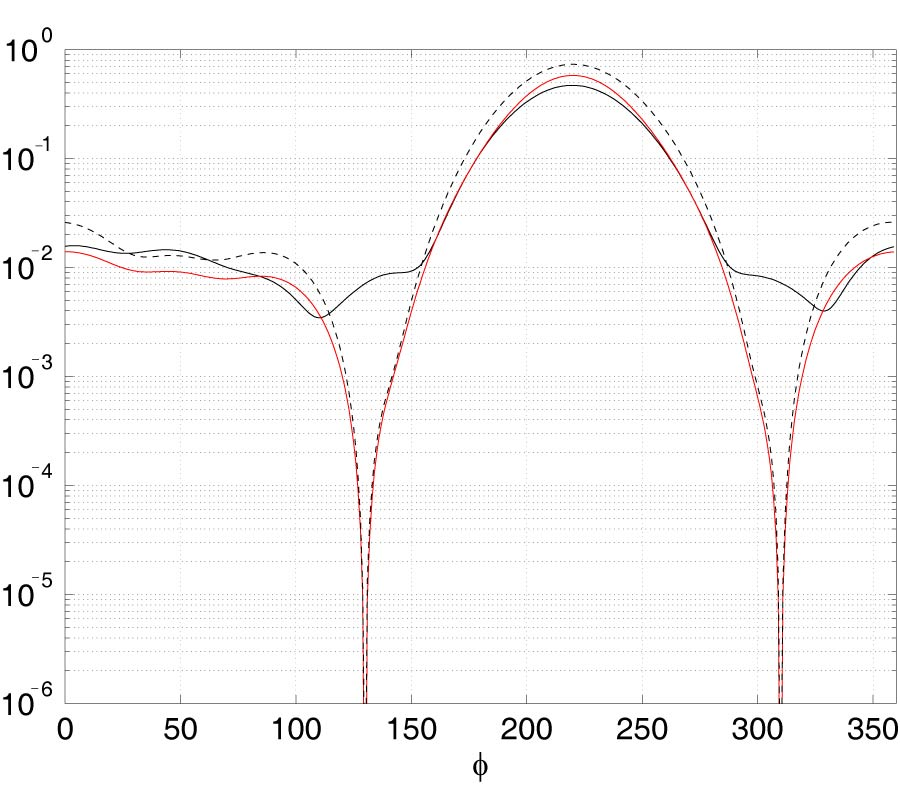# Vol. 46

Front:[PDF file] Back:[PDF file]
Latest Volume
All Volumes
All Issues
2012-11-24

#### Solution of the Electromagnetic Scattering Problem from an Electrically Large Random Dielectric Medium

By Bruno Stupfel and Mikael Picard
Progress In Electromagnetics Research B, Vol. 46, 79-100, 2013
doi:10.2528/PIERB12100804

## Abstract

The time-harmonic electromagnetic scattering problem from a random inhomogeneous dielectric medium (here a turbulent plasma wake created by the atmospheric reentry of a vehicle) is considered. The electronic density of the plasma, that gives rise to its dielectric permittivity, has a fluctuating part εf (r), the variance and correlation function of which are known a priori. Because the electrical dimensions of the wake can be very large, the numerical solution of Maxwell's equations via a full-wave calculation performed with a boundary element and finite element method is prohibitive when statistical quantities such as the mean Radar Cross Section (RCS) and its variance are required, that necessitate a large number of random realizations. To remedy this difficulty, two approximations are considered and illustrated for a 2D scattering problem. First, a Mie series approach is adopted where the medium is discretized with small disks, thus reducing considerably the number of unknowns for a given random realization of εf (r), and a domain decomposition method is proposed to further reduce the complexity of the numerical solution of the corresponding system. Second, the statistical mean and the variance of the RCS are derived in closed-form from the Born approximation and yield accurate results when, as expected, the statistical mean of the relative dielectric permittivity is close to unity and |εf (r)| is small. Conversely, it is shown how these expressions can be used to validate the results obtained with the Mie series approximation. Numerical examples are presented that illustrate the potentialities of these techniques.

## Citation

Bruno Stupfel and Mikael Picard, "Solution of the Electromagnetic Scattering Problem from an Electrically Large Random Dielectric Medium," Progress In Electromagnetics Research B, Vol. 46, 79-100, 2013.
doi:10.2528/PIERB12100804
http://www.jpier.org/PIERB/pier.php?paper=12100804

## References

1. Ishimaru, A., Wave Propagation and Scattering in Random Media, Academic Press, New York , 1974.

2. Finson, M. L., "Hypersonic wakes aerodynamics at high reynolds numbers," AIAA Journal, Vol. 11, No. 8, 1137-1145, 1973.
doi:10.2514/3.50559

3. Levensteins, Z. J. and M. V. Krumins, "Aerodynamic characteristics of hypersonic wakes," AIAA Journal, Vol. 5, No. 9, 1596-1602, 1967.
doi:10.2514/3.4256

4. Slattery, R. E. and W. G. Clay, "The turbulent wakes of hypersonic bodies," 17th Annual Meeting of the American Rocket Society, 1962.

5. Stupfel, B., "A hybrid finite element and integral equation domain decomposition method for the solution of the 3-D scattering problem," J. Comp. Phys., Vol. 172, 451-471, 2001.
doi:10.1006/jcph.2001.6814

6. Vouvakis, M. N., K. Zhao, S. M. Seo, and J. F. Lee, "A domain decomposition approach for non-conformal couplings between finite and boundary elements for unbounded electromagnetic problems in R3," J. Comp. Phys., Vol. 225, 975-994, 2007.
doi:10.1016/j.jcp.2007.01.014

7. Li, Y. J. and J. M. Jin, "Parallel implementation of the FETI-DPEM algorithm for general 3D electromagnetic simulations," J. Comp. Phys., Vol. 228, 3255-3267, 2009.
doi:10.1016/j.jcp.2009.01.029

8. Peng, Z. and J. F. Lee, "Non-conformal domain decomposition method with second order transmission conditions for time-harmonic electromagnetics," J. Comp. Phys., Vol. 229, 5615-5629, 2010.
doi:10.1016/j.jcp.2010.03.049

9. Berenger, J. P., "Numerical reflection from FDTD-PMLs: A comparison of the split PML with the unsplit and CFS PMLs," IEEE Trans. on Antennas and Propagat., Vol. 50, No. 3, 258-265, 2002.
doi:10.1109/8.999615

10. Chen, Q., M. Katsurai, and P. Aoyagi, "An FDTD formulation for dispersive media using a current density," IEEE Trans. on Antennas and Propagat., Vol. 46, No. 10, 1739-1745, 1998.
doi:10.1109/8.736632

11. Kong, J. A., Electromagnetic Wave Theory, John Wiley and Sons, 1986.

12. Jean, M., Diffraction D'une Onde Electromagnetique Par Un Plasma Turbulent: Application Au Calcul De La Surface Equivalente Radar, These de l'Universite Bordeaux I, 1995.

13. Tsang, L., C. E. Mandt, and K. H. Ding, "Monte Carlo simulations of the extinction rate of dense media with randomly distributed dielectric spheres based on solutions of Maxwell equations," Optics Lett., Vol. 17, No. 5, 314-316, 1992.
doi:10.1364/OL.17.000314

14. Elsherbeni, A. Z., M. Hamid, and G. Tian, "Iterative scattering of a gaussian beam by an array of circular conducting and dielectric cylinders," Journal of Electromagnetic Waves and Applications, Vol. 7, No. 10, 1323-1342, 1993.
doi:10.1163/156939393X00507

15. Elsherbeni, A. Z. and A. A. Kishk, "Modeling of cylindrical objects by circular dielectric and conducting cylinders," IEEE Trans. on Antennas and Propagat., Vol. 40, No. 1, 96-99, 1992.
doi:10.1109/8.123363

16. Lin, J. H. and W. C. Chew, "BiCG-FFT T-matrix method for solving for the scattering solution from inhomogeneous bodies," IEEE Trans. on Antennas and Propagat., Vol. 44, No. 7, 1150-1155, 1996.

17. Le Ravalec, M., B. Noetinger, and L. Y. Hu, "The FFT moving average (FFT-MA) generator: An e±cient numerical method for generating and conditionning Gaussian simulations," Mathematical Geology, Vol. 32, No. 6, 701-723, 2000.
doi:10.1023/A:1007542406333

18. Bardon, M., "Etude theorique Et Experimentale De La Polarisation Du Rayonnement Di®use Par Une Surface Rugueuse. Application A La Detection De Rayures,", These de l'Ecole Centrale Paris, 2003.

19. Gradshteyn, I. S. and I. M. Ryzhik, Table of Integrals, Series and Products, Academic Press, 1980.

20. Abramowitz, M. and I. E. Stegun, Handbook of Mathematical Functions, 1972.

21. Bonnemason, P., R. Le Martret, and B. Stupfel, "Oblique incidence scattering by large, coated cylinders of arbitrary cross-section," URSI Digest of the Joint Symposia IEEE-APS/URSI/NEM, Vol. 26, Chicago, July 1992.

22. Chew, W. C., "Recurrence relations for 3D scalar addition theorem," Journal of Electromagnetic Waves and Applications, Vol. 6, No. 1-4, 133-142, 1992.
doi:10.1163/156939392X01075

23. Chew, W. C. and Y. M. Wang, "Efficient way to compute the vector addition theorem," Journal of Electromagnetic Waves and Applications, Vol. 7, No. 5, 651-665, 1993.
doi:10.1163/156939393X00787